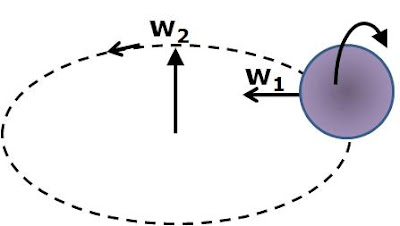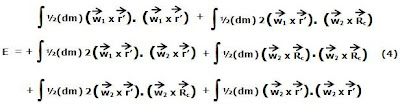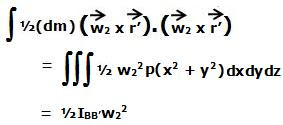## Sunday, February 8, 2009

### Irodov Problem 1.267The motion of any point in the sphere is a combination (sum) of two kinds of rotational motions, i) its rotation about the axis AA' (at a distance R from its center) as it moves in a circle on the plane with angular velocity w2 and ii) its rotation about the axis XX' (passing through its center) with an angular velocity w1. Since the tangential velocity of the sphere's center is v, we have,Under vector representation, angular velocity w2 is directed along the +ive z-axis while w1 is directed radially inwards always pointing towards the axis AA'.

We can solve the problem in two ways, i) using basic principles ii) using tensor notation. The tensor notation is much simpler to use and more generic and can be used to solve for more complex systems. I will solve using both these methods.Solution using basic principles: Consider an infinitesimally small piece of the sphere dm located at radius vector r' from the center of the sphere O. Further, suppose that Rc is the vector location of the center of the sphere with the point of intersection of XX' and AA' - O'.

The velocity vector v' of this infinitesimally small piece of mass is the sum of two components, i) the v1 velocity induced due to the sphere's roation about the axis XX' at rate w1 and ii) the velocity induced due to the sphere's rotational motion about the axis AA' at rate w2. This is given by,The kinetic energy dE of the infinitesimally small mass dm is given by,The total kinetic energy can be obtained by integrating over the entire sphere by varying r' to span all points in the sphere.Now let us examine each of these terms carefully. If the density of the sphere is p, then dm = pdxdydz. Then,Here, x,y,z, correspond to the x,y,z coordinates with BB' as the z-axis, XX' as the x-axis and origin as O. Ixx' is the moment of inertia of the sphere about the axis XX'.
Similarly,Now,is a constant and so we have,Now evaluating the rest of the terms,Hence, we have,Here, IAA' the moment of inertia of the sphere about the axis AA'. We know that (see list of moment of intertias),The moment of inertia of the sphere about axis AA' can be found using the parallel axis theorem as,Thus, the total kinetic energy of the sphere is given by,Solution using tensors: In the tensor world, the moment of inertia tensor I is a 3x3 matrix - a tensor of rank 2. The kinetic energy of a body rotating with an angular velocity vector w ( 3x1 matrix) is then given by.

Consider an instant when the axis XX' is oriented along the x-axis. Then consider the coordinate system with XX' and AA' as the x and z axes with y axis perpendicular to both of them and passing through their intersection. For this problem then using the parallel axis theorem,Thus, the kinetic energy of the system is given by.